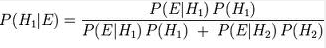## Thursday, April 18, 2013

### Summary of Findings (Green Team): Bayesian Analysis (3.75 out of 5 Stars)

Game Theory
Bayesian Analysis
Rating (3.75 out of 5 Stars)

Note: This post represents the synthesis of the thoughts, procedures and experiences of others as represented in the 8 articles read in advance (see previous posts) and the discussion among the students and instructor during the Advanced Analytic Techniques class at Mercyhurst University in April 2013 regarding Bayesian Analysis specifically. This technique was evaluated based on its overall validity, simplicity, flexibility and its ability to effectively use unstructured data.

Description:
Bayesian analysis, developed by Thomas Bayes, is a statistical approach that deals with using prior knowledge as well as updated information, separating the Bayesian approach from frequentist statistics. Bayesian analysis makes the analysis process iterative, allowing for more information to be added as it is learned or deemed relevant. According to Hubbard (2010), Bayesian theorem is a relationship of probabilities and conditional probabilities, or the chance of something given a certain condition (p.178-79). Bayesian analysis allows analysts to calculate probabilities or make estimates in terms of certain base assumptions as well as new developments. This is especially important in the intelligence field as analysts should be able to include new signals or indicators into their analysis to update estimates, further reducing their levels of uncertainty.

Strengths:
• Most helpful in situations in which your initial estimate is at either end of the spectrum
• Helps analysts to combat emotional/irrational estimates
• Particularly effective when analyzed in relation to the probability that was present prior to the addition of the newest piece of evidence.
• Has the potential to be applied across multiple disciplines, as evidenced more frequently in recent academic journals
Weaknesses:
• Complex, difficult to learn without a statistics background
• The volume of evidence makes it difficult to continuously evaluate the information
• No set way to decide what constitutes evidence
• Unable to know what is important at the moment, particularly with real-world situations
• Assumes that each piece of evidence is worth the same weight
• Is less useful in intelligence applications when probabilities are initially determined to be 50/50

How-To:
1. Start with a rudimentary estimation for the likelihood of an event occurring
2. Take evidence and apply/use probabilities for potential outcomes
3. Follow the formula whereby P is the probability, E is the event of interest, H is the factor likelihood, and E|H is the probability of the event of interest given the factor occurred in conjunction to it.4. Do the math to find the probability percentage.
5. This process can be repeated as additional information is given/discovered in order to improve the probability estimate.

Personal Application of Technique:
The class was tasked with finding out the likelihood that an individual, Bob, drove to work based on the fact that he was late. Bob can choose between three different ways to get to work: car, bus, or commuter train. If he takes his car, there is a 50% chance that he will be late. The bus has a 20% chance of making him late while the train has a 1% chance of making him late.

The first question that the class was given was to find out the likelihood that Bob drove to work based on the probability that Bob chose evenly between the three choices. Using Bayesian analysis, the class came up the a 70.4% probability that Bob drove to work that day.

The second question added new information about Bob’s normal transportation habits. Bob’s co-worker knew that Bob almost always takes the commuter train, never takes the the bus, and takes the car 10% of the time. With this new information, the class was able to update the probability that Bob drove to work to 84.75%.

This exercise demonstrated that the application of Bayesian Analysis is not always the most direct method to get to the probability of the situation in that with this particular case, the boss could specifically ask Bob how he got to work that morning. As individuals not seeking a degree in mathematics, there was a significant hesitation to apply the formula and present the findings.

Rating:  3.75 out of 5 stars
Note: The analysts feel this methodology has very strong benefits and is widely applicable, however, it is relatively weak in terms of its application to the intelligence community, particularly in the reliance on numerous factors which affect the utility.
For Further Information:
Hubbard, D. W. (2010). How to measure anything: Second edition. Hoboken, NJ: John Wiley & Sons, Inc.